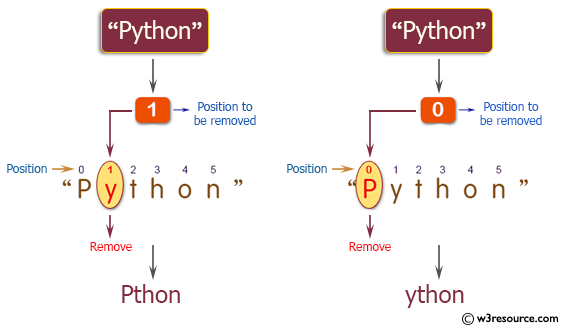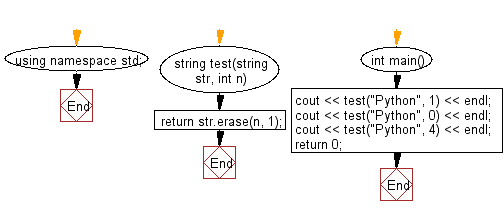﻿ C++ : Remove the character in a position of a given string# C++ Exercises: Remove the character in a given position of a given string

## C++ Basic Algorithm: Exercise-6 with Solution

Write a C++ program to remove the character in a given position of a given string. The given position will be in the range 0..string length -1 inclusive.

Sample Solution:

C++ Code :

``````#include <iostream>

using namespace std;

string test(string str, int n)
{
return str.erase(n, 1);
}

int main()
{
cout << test("Python", 1) << endl;
cout << test("Python", 0) << endl;
cout << test("Python", 4) << endl;
return 0;
}
``````

Sample Output:

```Pthon
ython
Pythn
```

Pictorial Presentation:Flowchart:C++ Code Editor:

Contribute your code and comments through Disqus.

What is the difficulty level of this exercise?

﻿